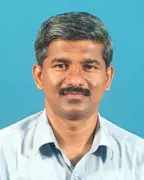X
Meshfree analysis of two-dimensional contaminant transport through unsaturated porous media using EFGM
Published in
2010
Volume: 26

Issue: 12
Pages: 1797 - 1816
Abstract
The development of numerical methods for modelling contaminant transport in the subsurface is of major importance in view of the numerous applications in the contaminant hydrogeology and geoenvironmental engineering. Conventional mesh-based numerical methods have some difficulties when dealing with contaminant transport problems wherein high advective velocities, low dispersivities and/or high contrast in dispersivity are involved. The present study aims at contributing to the numerical study of twodimensional contaminant transport thorough unsaturated porous media using meshfree technique, namely element-free Galerkin method (EFGM). In the EFGM, an approximate solution is constructed entirely in terms of a set of nodes and no characterization of the interrelationship of the nodes is needed. The EFGM employs moving least squares approximants to approximate the function and uses the Lagrange multiplier method for imposing the essential boundary conditions. van Genuchten model is used for describing the hydrodynamic properties of the unsaturated porous media. MATLAB code is developed to obtain the numerical solution. Three numerical examples are presented and the results are compared with those obtained from the finite element method. The EFGM has generated stable and convergent results for advection-dominated transport problems. © 2009 John Wiley & Sons, Ltd.
Journal International Journal for Numerical Methods in Biomedical Engineering 20407939 No
Authors (1)
•Concepts (28)
••APPROXIMANTS
•Approximate solution
•Contaminant transport
•DISPERSIVITY
•Element-free galerkin method
•ESSENTIAL BOUNDARY CONDITIONS
•Geoenvironmental engineering
•HIGH CONTRAST
•HYDRODYNAMIC PROPERTIES
•LAGRANGE MULTIPLIER METHOD
•Matlab code
•MESHFREE ANALYSIS
•MESHFREE TECHNIQUE
•MODELLING CONTAMINANTS
•Moving least squares
•Numerical example
•Numerical solution
•TRANSPORT PROBLEMS
•UNSATURATED POROUS MEDIA
•VAN GENUCHTEN MODEL
•Computational mechanics
•Finite element method
•Groundwater
•Hydrogeology
•Lagrange multipliers
•Numerical methods
•Porous materials